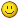# Severe Problem with calculated Weight and volume

Dear Concern,

Today I am facing a severe problem with the calculated weight and volume, anyone also faced this panic situation.

How to get rid of these weight and volume big numbers.?

Any help would be much appreciated.
Kind Regards,

Hi,

With only that little information it will be difficult to help.

Then Which information I can show here, let me know I will post over here

Hellosince it upgrade to dolibarr 10 (from 6) all my weight are in wrong unit (kg instead of g)

Problem comes from the function getTotalWeightVolume in core/class/commonobject.class.php uses unit index instead of dictionary ‘scale’ to convert values.
(issue filed as https://github.com/Dolibarr/dolibarr/issues/11861)
If you feel like changing the code, find the lines

``````                    \$weightUnit=0;
\$volumeUnit=0;
if (! empty(\$weight_units)) \$weightUnit = \$weight_units;
if (! empty(\$volume_units)) \$volumeUnit = \$volume_units;

if (empty(\$totalWeight)) \$totalWeight=0;  // Avoid warning because \$totalWeight is ''
if (empty(\$totalVolume)) \$totalVolume=0;  // Avoid warning because \$totalVolume is ''
``````

and change them to :
\$weightUnit=0;
\$volumeUnit=0;
if (! empty(\$weight_units)) \$weightUnit = getDictvalue(MAIN_DB_PREFIX.‘c_units’, ‘scale’, \$weight_units);
if (! empty(\$volume_units)) \$volumeUnit = getDictvalue(MAIN_DB_PREFIX.‘c_units’, ‘scale’, \$volume_units);

``````                    if (empty(\$totalWeight)) \$totalWeight=0;  // Avoid warning because \$totalWeight is ''
if (empty(\$totalVolume)) \$totalVolume=0;  // Avoid warning because \$totalVolume is ''
``````

Kindest regards,
Marc